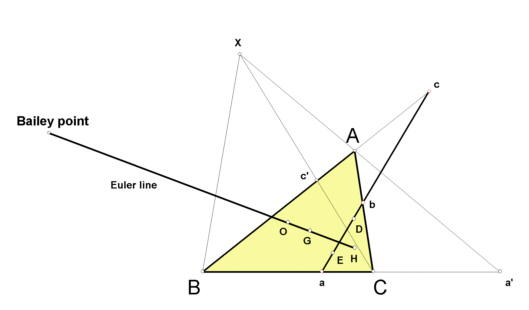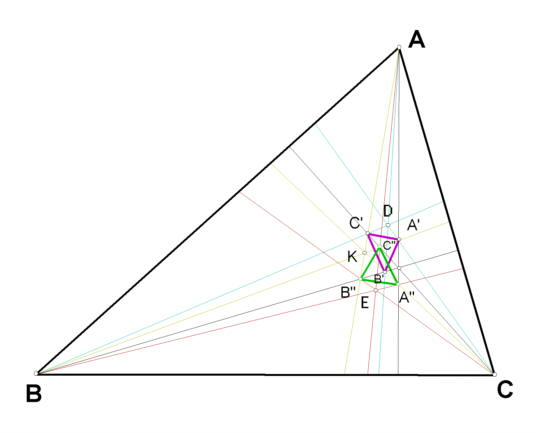# BAILEY POINT

Late in 1998 a new point on the Euler line was found and named in honor of V. C. Bailey, Professor Emeritus of Mathematics, University of Evansville, on the occasion of his ninety-fourth birthday.

#To construct the Bailey point, let L be the altitude through vertex A, let K be the symmedian point, and let M be the line BK Let A' be the point where L meets M. Construct B' likewise, using the B-altitude and line CK; and construct C' likewise, using the C-altitude and line AK. (In the figure below, L and M appear but are not labeled.)

Now let N be the line through vertex C and point K. Construct points A", B", C" following the method given above for A', B', C'.

The triangles A'B'C' and A"B"C" are, notably, each triply perspective to the other and each triply perspective to triangle ABC. These relationships are indicated by the figure:

#Lines AB', BC', CA' concur in a point D, and lines AC", BA", CB" concur in a point E. The isogonal conjugate of the trilinear pole of line DE is the Bailey point.

Trilinears for the Bailey point are

(csc A)(sin 2B sin 2C - sin 2A sin 2A) :
(csc B)(sin 2C sin 2A - sin 2B sin 2B) :
(csc C)(sin 2A sin 2B - sin 2C sin 2C).

The Bailey point provides a good example of a kind of conjugate that seems to be new. If

U = u : v : w and X = x : y : z

are points for which not all three of the coordinates of

vwx^2 - yzu^2 : wuy^2 - zxv^2 : uvz^2 - xyw^2

are zero, then this point defines the U-diamond conjugate of X. Diamond conjugates and the Bailey point are discussed in

Clark Kimberling, "Diamond Conjugates," submitted (1999).

Preprints are available - just drop me a line at ck6@evansville.edu .

(For a quick lesson on trilinears, click on Trilinear Coordinates.)
Euler line
Triangle Centers